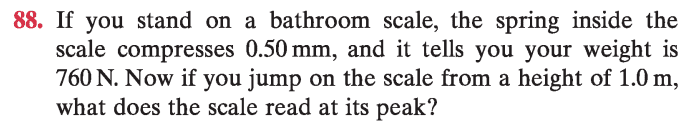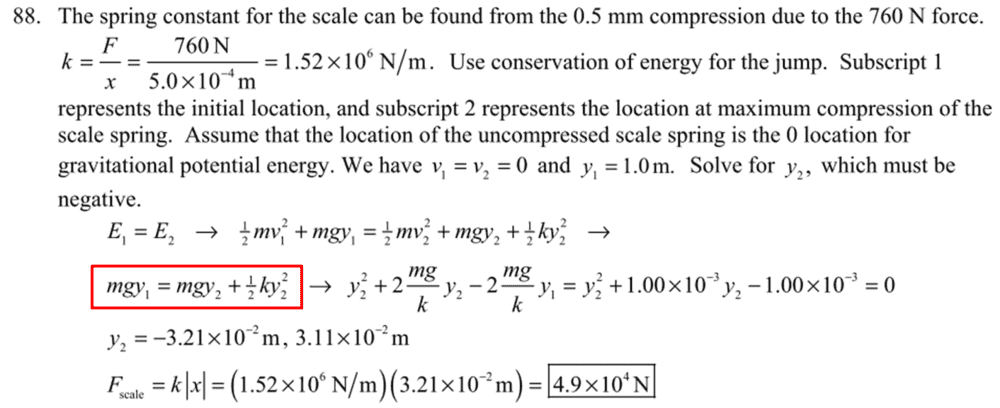# Using Energy Conservation to solve this problem: Jumping on a spring scale

r12214001
Homework Statement:
my cal:760(1+X)=0.5kX²
Relevant Equations:
760(1+X)=0.5kX²
I suppose spring compression to be X when jumpping from 1m. Therefore gravational potential mgh=760(1+X) and my cal:760(1+X)=0.5kX²
why the solution manual state that 760=0.5kX²+760XLast edited:

Homework Helper
I suppose spring compression to be X when jumpping from 1m. Therefore gravational potential mgh=760(1+X) and my cal:760(1+X)=0.5kX²
why the solution manual state that 760=0.5kX²-760X
Algebra.

Start with$$760(1+X)=0.5kX^2$$ and subtract ##760X## from both sides to get $$760(1+X)-760X=0.5kX^2-760X$$Now on the left hand side notice that you can cancel that 760X and get $$760=0.5kX^2-760X$$

r12214001
Algebra.

Start with$$760(1+X)=0.5kX^2$$ and subtract ##760X## from both sides to get $$760(1+X)-760X=0.5kX^2-760X$$Now on the left hand side notice that you can cancel that 760X and get $$760=0.5kX^2-760X$$
typing error
solution manual state that 760=0.5kX²+760X

Homework Helper
Gold Member
2022 Award
typing error
solution manual state that 760=0.5kX²+760X
The solution manual took ##X## to be negative.

•